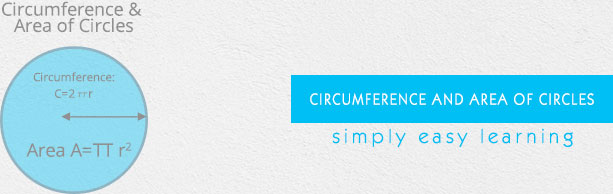# Circumference and Area of Circles

This tutorial provides comprehensive coverage of Circumference and Area of Circles based on Common Core (CCSS) and State Standards and its prerequisites. Students can navigate learning paths based on their level of readiness. Institutional users may customize the scope and sequence to meet curricular needs. This simple tutorial uses appropriate examples to help you understand Circumference and Area of Circles in a general and quick way.

# Audience

This tutorial has been prepared for beginners to help them understand the basics of Circumference and Area of Circles. After completing this tutorial, you will find yourself at a moderate level of expertise in Circumference and Area of Circles, from where you can advance further.

# Prerequisites

Before proceeding with this tutorial, you need a basic knowledge of elementary math concepts such as number sense, basic arithmetic operations, whole numbers, fractions, decimals, geometric concepts like point, line, curve, length, area, plane shapes and so on.

Advertisements